# Division - 7th grade (12y) - math problems

#### Number of problems found: 255

• Shirt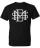Mrs. Vítková bought each of his three children the same shirt paid CZK 1,000. Saleswoman she returned 568,60 CZK. What was the price of one shirt?
• Simple equation 2Find X in this simple equation: X/9 = 96/108
• LCMWhat is the least common multiple of 5, 50, 14?
• Result and remainderAfter dividing the unknown number by the number 23, the quotient 11 and the remainder 4 are formed. Find an unknown number.
• Twenty-fiveHow many three-digit natural numbers are divisible by 25?
• Percent calculationCalculate 51% if 99% is 79.
• Bicycle wheel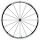Bicycle wheel diameter is 62 cm. How many times turns the bicycle on the road 1 km long?
• Number ratio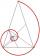Calculate two positive numbers that its ratio is 6:6 and difference was 0.
• Sum of dividendIf X is divided separately 2.63 and 1.40. And the results of the individual divisions are added to give a result less than X. What is X?
• DivisibilityWrite all the integers x divisible by seven and eight at the same time for which the following applies: 100
• SymbolsIf 2*3 = 60 ; 3*4 = 120 and 4*5 = 200, what is 2*5?
• CustomerA customer purchase three (3) writing pads from a store. She receive k9.70 change from a k10.00 note . How much was it's writing pad cost?
• Divide 5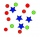Divide 288 in the following ratio 3 : 4 : 5
• Ounce749 calories in a 7 - ounce serving . What is the unit rate?
• Decomposition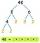Make decomposition using prime numbers of number 155. Result write as prime factors (all, even multiple)
• Unknown numberIf I reduced the sum of the numbers 70 and the unknown number three times, I would get 100. what is the unknown number?
• Discount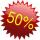Product has been discounted twice by 19%. What is the total discount given?
• Money split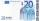Anton and Ferko got 2,500 euros together. The amount was split in 1:4 ratio. How much did each get?
• MPG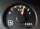252 miles a week fills up twice a week. Each fill is 38 gallons. What is gas mileage of the car?
• Simple sequence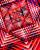Continue with this series of numbers: 1792,448, 112, _, _

Do you have an interesting mathematical word problem that you can't solve it? Submit a math problem, and we can try to solve it.

We will send a solution to your e-mail address. Solved examples are also published here. Please enter the e-mail correctly and check whether you don't have a full mailbox.

Please do not submit problems from current active competitions such as Mathematical Olympiad, correspondence seminars etc...